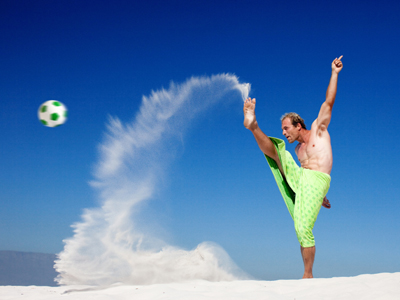This soccer ball is a sphere and is therefore NOT a polyhedron.

# Shapes - Polyhedra

This Math quiz is called 'Shapes - Polyhedra' and it has been written by teachers to help you if you are studying the subject at middle school. Playing educational quizzes is a fabulous way to learn if you are in the 6th, 7th or 8th grade - aged 11 to 14.

It costs only \$12.50 per month to play this quiz and over 3,500 others that help you with your school work. You can subscribe on the page at Join Us

In Math, a 3-D (solid) shape made by fitting polygons together is called a polyhedron. You have already met some polyhedra, e.g. cuboids. A regular polyhedron is formed from regular polygons all the same size and shape.

1.
Which of these shapes is not a polyhedron?
Cube
Pyramid
Sphere
Triangular prism
Polyhedra have straight edges and flat surfaces
2.
The polygons forming the surface of a polyhedron are called .......
areas
edges
faces
sides
3.
Where the straight sides of two polygons meet on the surface of a polyhedron they form a(n) .......
area
edge
line
side
4.
The place on the surface of a polygon where several edges meet is called a .......
corner
dent
point
vertex
5.
What is the name of the regular polyhedron in which four equilateral triangles meet at each vertex?
Hexahedron
Icosahedron
Octahedron
Tetrahedron
Imagine two square-based pyramids stuck together
6.
If you add the number of faces of a polyhedron to the number of its vertices the result is always .......
equal to the number of edges
2 more than the number of edges
2 less than the number of edges
1 more than the number of edges
V + F = E + 2 is a formula discovered by the Swiss mathematician Leonhard Euler
7.
How many edges has a pyramid with a hexagonal base?
6
8
10
12
Use Euler's formula with F = 7 and V = 7
8.
How many faces has the regular polyhedron made with pentagons?
5
10
12
15
This is called a dodecahedron
9.
A polyhedron with four rectangles or squares meeting at each vertex is called .......
a cuboid
a parallelepiped
a trapezoid
impossible
When four right angles meet at a point they completely fill the flat space so they cannot be folded into a solid
10.
A polyhedron formed from rectangles set at right-angles to a polygonal base and top is called a .......
parallel
prism
prune
pyramid
The cross section of a prism is the same shape and size along its whole length
Author:  Frank Evans